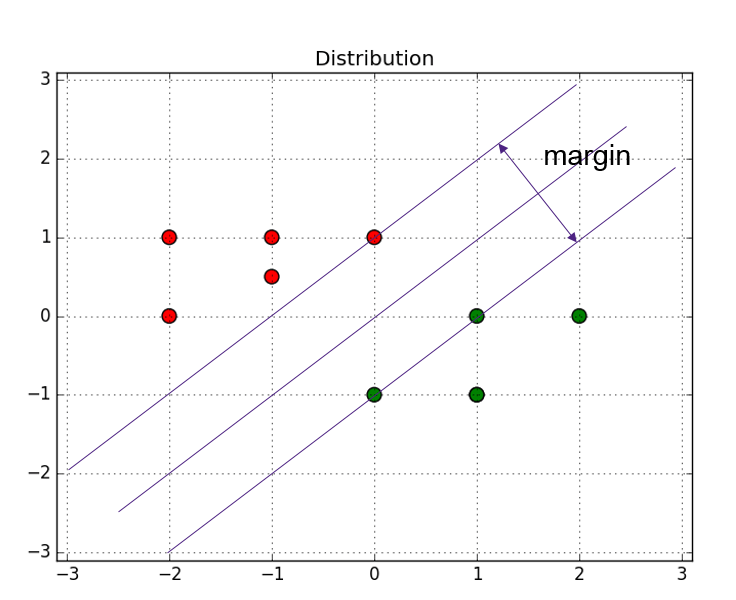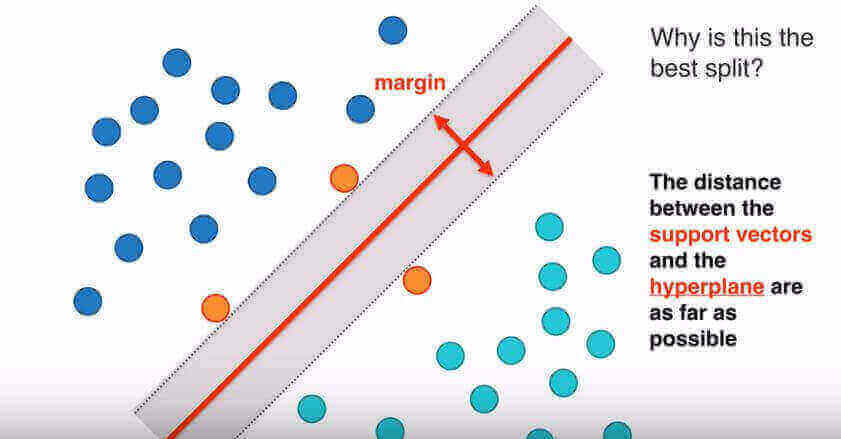#### 支持向量机（SVM）里的支持向量是什么意思

统计/机器学习 监督式学习    浏览次数：12721        分享

5MeganC   2017-02-27 16:01

##### 6个回答
19SofaSofa数据科学社区DS面试题库 DS面经crazy_lau   2017-09-21 15:04

6

SVM可以用下面这个图表示。SofaSofa数据科学社区DS面试题库 DS面经sasa   2018-01-19 04:13

4

SofaSofa数据科学社区DS面试题库 DS面经CE_PAUL   2018-06-18 20:23

3

soft margin的SVM的loss function

$$l(w)=\frac{1}{n}\sum_{i=1}^{n}max(0,1-y_i(w^Tx_i-b))+\lambda||w||^2$$

support vectors是$1-y_i(w^Tx_i-b)\geq 0$的点，或者说在loss function中有贡献的点。具体找support vectors，要用dual problem方法。假设已经找出所有support vectors，有

$$l(w)=\frac{1}{n}\sum_{i\in S}(1-y_i(w^Tx_i-b))+\lambda||w||^2$$

$$\dfrac{dl(w)}{dw}=\frac{1}{n}\sum_{i\in S}(-y_ix_i)+\frac{\lambda}{2}w=0$$

$$w=\frac{2}{n\lambda}\sum_{i\in S}(y_ix_i)$$

$$w=\frac{2}{n\lambda}\sum_{i\in S}(x_i')$$

SofaSofa数据科学社区DS面试题库 DS面经Zealing   2018-10-09 11:08

2

SofaSofa数据科学社区DS面试题库 DS面经图不二   2017-11-21 12:25

1SofaSofa数据科学社区DS面试题库 DS面经ZhuangChong   2018-06-13 10:05相关主题